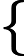Study of mathematics online.
Study math with us and make sure that "Mathematics is easy!"

# Formulas and properties of nth root

Definition
The nth root of the number a is a number b, n-th power is equal to a
.
The following properties hold for nth root (a > 0, b > 0 and n, m, k is natural numbers):
1. na b = na · nb

2. $\sqrt[n]{\frac{a}{b}}=\frac{\sqrt[n]{a}}{\sqrt[n]{b}}$

3. $(\sqrt[n]{a})^k=\sqrt[n]{a^k}$

4. $\sqrt[n]{\sqrt[m]{a}}=\sqrt[nm]{a}$

5. $\sqrt[nk]{a^k}=\sqrt[n]{a}$

6. $\sqrt[n·k]{a^{m·k}}=(\sqrt[n]{a^m})$

7.  $\sqrt[n]{a^n}=$|a| if n – even a if n – odd

8. For any a and b, such that 0 ≤ ab truly following inequality:
n a n b